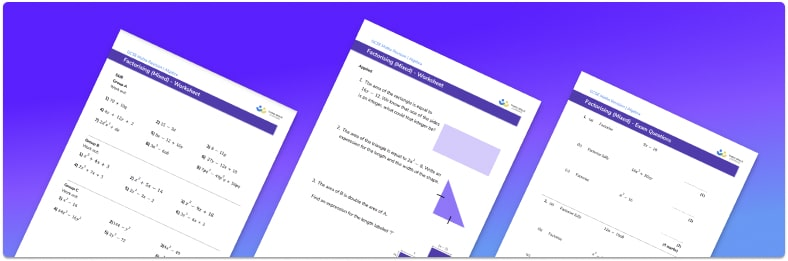# Factorising Worksheet• Section 1 of the factorising worksheet contains 21 skills-based factorising questions, in 3 groups to support differentiation
• Section 2 contains 3 applied factorising questions with a mix of worded problems and deeper problem solving questions
• Section 3 contains 2 foundation and higher level GCSE exam questions to practise factorising algebraic expressions
• Answers and a mark scheme for all questions are provided
• Questions follow variation theory with plenty of opportunities for students to work independently at their own level
• All skills based and GCSE questions created by fully qualified expert secondary maths teachers
• Suitable for GCSE maths revision for AQA, OCR and Edexcel exam boards

• This field is for validation purposes and should be left unchanged.

You can unsubscribe at any time (each email we send will contain an easy way to unsubscribe). To find out more about how we use your data, see our privacy policy.

### Factorising at a glance

Factorising is the inverse of expanding brackets. It involves rewriting an expression so that it becomes the product of two (or more) terms in brackets.

The topic begins with factorising binomials (polynomials with 2 terms) into a single bracket using common factors. This involves skills such as comparing the coefficients of the two terms and considering their highest common factor (hcf). The highest common factor may be a number, a variable (a letter) or both. Both terms may have indices, so an understanding of indices can be very useful.

The topic moves onto factorising trinomials (polynomials with 3 terms) such as quadratic expressions. This leads to rewriting the trinomial as a product of two factors, each with its own set of brackets. This is often referred to as factorising quadratics with double brackets. This can be done by looking at the constant term and finding its factor pairs and calculating which pair sum to make the coefficient of the x term. We can use factorising quadratics as a method for solving quadratic equations.

When a binomial expression involves a square term being subtracted from another square term, we can factorise it by using the difference of two squares method. This results in double brackets. Knowledge of square numbers is useful when factorising using this method.

Looking forward, students can progress with more factorising worksheets to additional algebra worksheets, for example a solving equations worksheet, simultaneous equations worksheet, or a rearranging equations worksheet.For more teaching and learning support on Algebra our GCSE maths lessons provide step by step support for all GCSE maths concepts.

## Do you have KS4 students who need more focused attention to succeed at GCSE?There will be students in your class who require individual attention to help them succeed in their maths GCSEs. In a class of 30, it’s not always easy to provide.

Help your students feel confident with exam-style questions and the strategies they’ll need to answer them correctly with our dedicated GCSE maths revision programme.

Lessons are selected to provide support where each student needs it most, and specially-trained GCSE maths tutors adapt the pitch and pace of each lesson. This ensures a personalised revision programme that raises grades and boosts confidence.

Find out more